# How to Count Dates of Given Year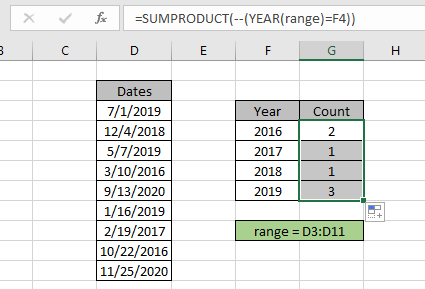In this article, we will learn how to count dates of a particular year in a range of dates.

In simple words, while working with dates, sometimes we need to count the cells where dates are from a given year.

How to solve the problem?

For this article we will be required to use the SUMPRODUCT function. If we are given dates in a range of data and a specific year value, we need to count the date values where the date belongs the specific given year.

Generic formula:

= SUMPRODUCT ( -- ( YEAR(range) = year ) )

range : date values given in as range
year : year value given as cell reference.

Example:
So, let's test this formula via running it on the example shown below.
Here we have the data records and we need to find the date values lying in a specific year or return the count rows where the date value lies within a given year.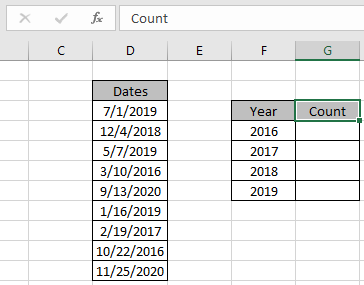Firstly, we need to define the named range for dates range as range. Just select the date values and type the name (range) for the range in the top left corner.

Now we will use the following formula to get a count of the dates which lay in year 2016.

Use the Formula:

= SUMPRODUCT ( -- ( YEAR ( range ) = F4 ) )

range: named range used for date values D3:D11.

F4: year value given as cell reference.

Explanation:

• YEAR ( range ) function extracts the year value from the date and matches it with given year and operator.

= SUMPRODUCT ( -- ( { 2019 ; 2018 ; 2019 ; 2016 ; 2020 ; 2019 ; 2017 ; 2016 ; 2020 } = 2016 ) )

• = operator matches it with a year value 2019 and returns an array of TRUE and FALSE values depending on the operation.

= SUMPRODUCT ( -- ( { FALSE ; FALSE ; FALSE ; TRUE ; FALSE ; FALSE ; FALSE ; TRUE ; FALSE } ) )

• -- operator used to convert TRUE value to 1 & False value to 0.

= SUMPRODUCT ( { 0 ; 0 ; 0 ; 1 ; 0 ; 0 ; 0 ; 1 ; 0 } )

• SUMPRODUCT function gets the sum of 1s in the returned array, which will be the count of required dates or rows.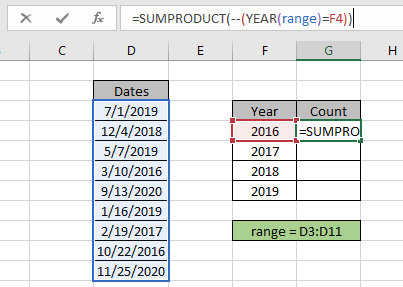Here the year value is given as cell reference and range is given as cell reference. Press Enter to get the count.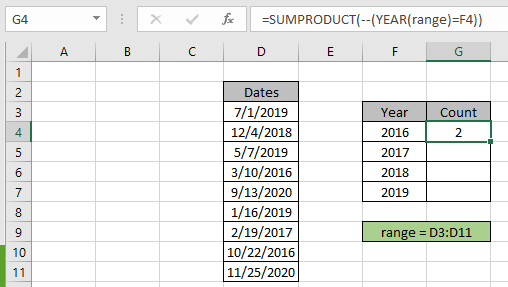As you can see the total date matches the year 2016 comes out to be 2. It means there are 2 date values or 2 rows where the year value equals to 2016.

Now copy and paste the formula to other cells using the CTRL + D or drag down option of Excel.As you can see in the above snapshot we obtain all the data values which lay in a specific year using the Excel formula. You can also obtain checks for the same using the excel filter option. Apply the filter to the Dates header and deselect the select all option and select the year option you need to filter.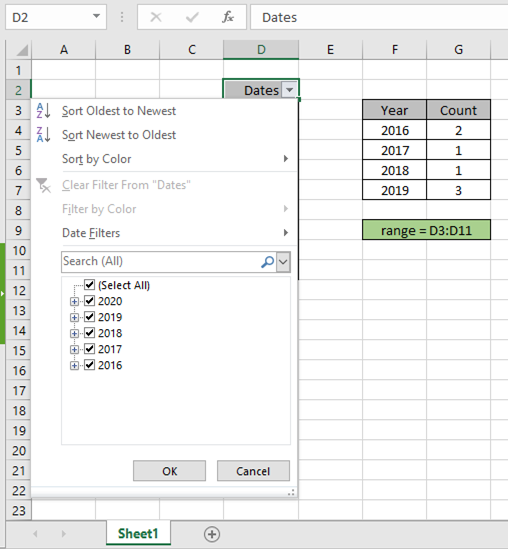The filter option returns 3 values as a result. Perform the same to other date values to get the count of dates or rows having criteria as given specific year.

Here are some observational notes shown below.

Notes:

1. The formula only works with numbers.
2. The formula only works when there are no duplicates on the lookup table.
3. The SUMPRODUCT function considers non- numeric values as 0s.
4. The SUMPRODUCT function considers logic value TRUE as 1 and False as 0.
5. The argument array must be of the same length as the function.

Hope this article about how to Return Count if with SUMPRODUCT in Excel is explanatory. Find more articles on SUMPRODUCT functions here. Please share your query below in the comment box. We will assist you.

Related Articles

How to use the SUMPRODUCT function in Excel: Returns the SUM after multiplication of values in multiple arrays in excel.

COUNTIFS with Dynamic Criteria Range : Count cells dependent on other cell values in Excel.

COUNTIFS Two Criteria Match : Count cells matching two different criteria on list in excel.

COUNTIFS With OR For Multiple Criteria : Count cells having multiple criteria match using the OR function.

The COUNTIFS Function in Excel : Count cells dependent on other cell values.

How to Use Countif in VBA in Microsoft Excel : Count cells using Visual Basic for Applications code.

How to use wildcards in excel : Count cells matching phrases using the wildcards in excel

Popular Articles

50 Excel Shortcut to Increase Your Productivity

Edit a dropdown list

Absolute reference in Excel

If with conditional formatting

e year 2019, select the year 2019 and click OK.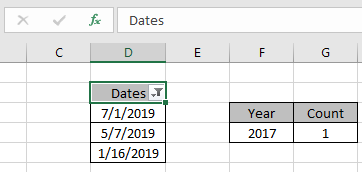Terms and Conditions of use

The applications/code on this site are distributed as is and without warranties or liability. In no event shall the owner of the copyrights, or the authors of the applications/code be liable for any loss of profit, any problems or any damage resulting from the use or evaluation of the applications/code.